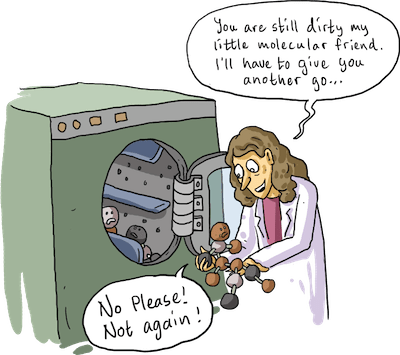# DIY Percent Composition

Alright so say you are a renowned chemist, but you have a flaw: you cannot figure out the percent composition of a certain compound! Before your hair falls out due to the agonizing stress, take a deep breath and we will go through percent composition step-by-step. Ask yourself: What exactly is percent composition?  Percent composition is the percentage by mass of each element in a compound. Why does this matter when dealing with chemistry? Percent composition is important because it shows how much a certain substance is comprised of one component or other. How do I figure this glorious aspect of chemistry out? Well lets go through a few steps.

Say I have H2O. What percent of this compound is Oxygen?

First, you find the molar mass of each of the atoms. Hydrogen is 1.01g and there’s 2 of them so that is 2.02 g. Oxygen’s mass is 16.00g grams. The total mass is 18.02 g.

*you can find the atomic masses on the periodic table. Just look for the atomic mass and plug it in!

When you think of percent composition try to always remember Part/Whole. Put the part you are trying to figure out on the top and the total mass. For our H2O example, since we are trying to find oxygen, put 16.00g/18.02g. That was the mass of the oxygen versus the total mass of the compound. Divide these and you should get .887 remember your significant digits like we talked about in the other blog! Try to keep them consistent.

Lastly, you are going to multiply the decimal you calculated by 100, which will give the percent. In this case oxygen is 88.7% of the compound H2O.

Why is this important? Well, one can use percent composition to figure out chemical formulas and determine the different amounts of elements in compounds which would be very useful in a trial and error  experiment.If you need extra help with percent composition, or you want to review more examples visit the site listed below.

http://www.chem.tamu.edu/class/majors/tutorialnotefiles/percentcomp.htm